• 做了一个项目需要把matlab下的m文件生成dll或者exe或者com组件在c/c++下使用。m文件中涉及到matlab中的神经网络函数 ，没有神经网络时候dll，exe，com都可以成功调用实习想要的结果，现在有神经网络的情况下我现在...
做了一个项目需要把matlab下的m文件生成dll或者exe或者com组件在c/c++下使用。m文件中涉及到matlab中的神经网络函数 ，没有神经网络时候dll，exe，com都可以成功调用实现想要的结果无论是画图还是计算，现在有神经网络函数的情况下我现在做到是都出现下面的问题。（test1是matlab的m文件包含了神经网络函数patternet）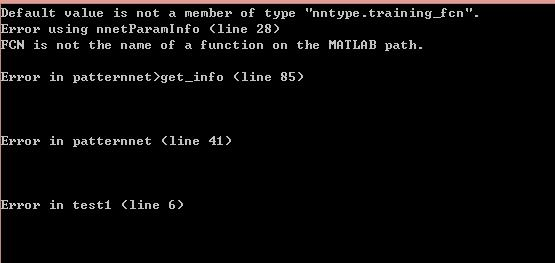总结：需要自行实现神经网络算法。
1 ,dll 的情况，使用用是matlab生成的libTest1.h,libTest1.lib,libTest1.dll三个文件
（1）libTestInitialize();
（2）mlfTest（params）;
（3）libTestTerminate();
2,exe的情况，使用matlab生成的exe，网上有很多方法。
3,com组件
这个文章
VC++和MATLAB的混合编程之神经网络工具箱
中提到可以调用matlab神经网络工具箱。
。生成com组件使用。。.bat注册COM组件，使用下面代码 调用函数，代码运行出现上面图片中错误。查原因是matlab的toolbox神经网络函数不能用。
#include "stdafx.h"
#include "stdio.h"
#include "testCom_idl_i.c"
#include "testCom_idl.h"
#include<objbase.h>
#include <iostream>
using namespace std;
#include<objbase.h>
int main(){
//exe :E:\\trainNet.exe
//parameters:D:\\autoextract\\fv.mat D:\\autoextract\\testdata\\ D:\\autoextract\\triandata\\
if (FAILED(CoInitialize(NULL)))
{
cout << "unable to initialize COM" << endl;
}
IClass1  * pIClass;//接口指针
HRESULT hr = CoCreateInstance(CLSID_Class1,
NULL,
CLSCTX_INPROC_SERVER,
IID_IClass1,
(void **)& pIClass);
if (SUCCEEDED(hr))
{
cout << "succssed" << endl;
VARIANT *net=NULL;
hr = pIClass->test1(1,net);//com中方法
cout << net << endl;

pIClass->Release();
}
CoUninitialize();
system("pause");
return 0;
}


展开全文• VS调用matlab生成dll 一、 编程环境  VS2012 ultimate  Matlab2012a 32位 二、 matlab程序转dll  1. matlab程序简单实例 % Myadd.m Function [result] = myadd(a,b) Result = a + b; end 2. 转dll步骤 ...
VS调用matlab生成的dll
一、 编程环境
VS2012 ultimate
Matlab2012a 32位
二、 matlab程序转dll
1. matlab程序简单实例
Result = a + b;
end
2. 转dll步骤
        命令行中输入：mbuild–setup，再选择编译的环境；
        生成多个文件，有用的文件为libname.lib、libname.h、libname.dll，切记libname.cpp
只是参考用的，不要加入到VS项目里面。
三、VS工程建立
1、包含库及链接库设置
右键项目-属性，在弹出的界面中：
在【C/C++选项】中：
        【包含目录】中添加：D：\matlab\extern\include;
        【库目录】中添加：D:\matlab\extern\lib\win32\microsoft;
在【链接器】选项中：
        【常规-附加库目录】中添加：D:\matlab\extern\lib\win32\microsoft;
注意：
2、调用matlab的dll程序实例
#include
using namespace std;
int main()
{
{
int aval = 6;
int bval = 3;
int result;
            //为变量分配内存空间
mwArray mwA(1,1,mxINT32_CLASS);
mwArray mwB(1,1,mxINT32_CLASS);
mwArray mwC(1,1,mxINT32_CLASS);
mwA.SetData(&aval,1);
mwB.SetData(&bval,1);

result = mwC.Get(1,1);

cout<<"result is "<<result<<endl;

//关闭mcr
mclTerminateApplication();
system("pause";
}
else
{
cout<<"initialize failed"<<endl;
}
return 0;

}
三、执行生成的exe
执行前，需要把libname.lib、libname.h、libname.dll放在exe所在文件夹。
四、参考链接
1、本文链接：
https://wenku.baidu.com/view/a7e … 7916888486b9b2.html
2、显式调用&隐式调用：https://www.jianshu.com/p/b4a2ce409aab
3、参考链接：https://www.cnblogs.com/jmliao/p/5575202.html


展开全文• matlab版本为 matlab R2016a 生成dll版本也是64位， 如果想32的dll,那就去把matlab的版本降下来！！ 首先绑定vs版本 我安装的是vs2013 分别输入 mex -setup 和 mbuild -setup 选择编译器 编写matlab函数 Add.m...
matlab版本为 matlab R2016a  生成dll版本也是64位， 如果想32的dll,那就去把matlab的版本降下来！！

首先绑定vs版本 我安装的是vs2013   分别输入 mex -setup  和 mbuild -setup 选择编译器r=a+b;
end

生成动态库：

生成的有.dll .lib .h .cpp 还要别的 用的就.dll .lib .h

添加配置文件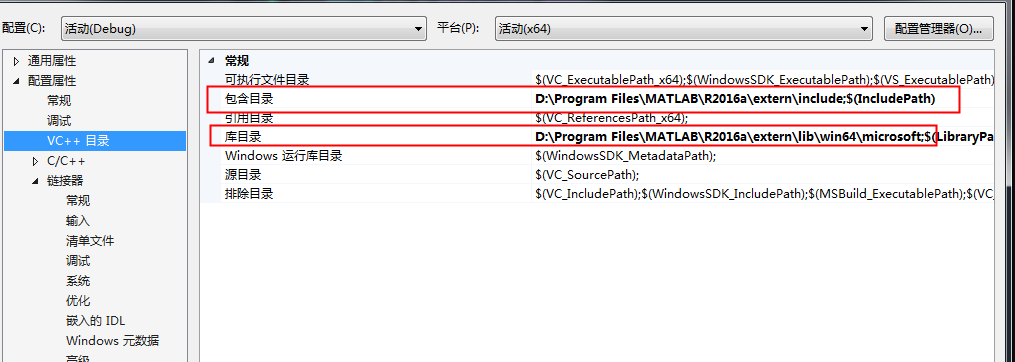配置环境变量：D:\Program Files\MATLAB\R2016a\bin\win64

添加链接器附加依赖项：

libmat.lib
libmex.lib
mclmcr.lib
mclmcrrt.lib
libemlrt.lib
libeng.lib
libfixedpoint.lib
libcovrt.lib

配置完毕

创建测试程序

#include <stdio.h>
#include <iostream>

using namespace std;

int main()
{
int jieguo = 0;
{
printf("初始化失败！");
exit(0);
}
printf("OK!\n");

mwArray result(1, 1, mxINT32_CLASS, mxREAL);
mwArray a(1, 1, mxINT32_CLASS, mxREAL);
//一行一列的矩阵
mwArray b(1, 1, mxINT32_CLASS, mxREAL);
//一行一列的矩阵
int data1 = 2; int data2 = 5;

a.SetData(&data1, 1);            //赋值
b.SetData(&data2, 1);

double d = result;
//获取结果
cout << "result=" << result << endl;
system("pause");
return 0;
}

嗯 这样就可以了

展开全文• 使用vs2008 sp1 调用 matlab2011b生成的dell，并且matlab中的函数调用包括.p文件，已经经过测试
• m语言生成dll库 在Qt Creator中使用c++库 疑问 m语言生成dll库 1. 首先编写一个简单的m函数test.m并保存 % test.m function ans = test(x,y) ans = x + y; end 2. 在matlab的命令行中输入deploytool并按回车...
目录

m语言生成dll库

在Qt Creator中使用c++库

疑问

m语言生成dll库

1. 首先编写一个简单的m函数test.m并保存

% test.m
function ans = test(x,y)
ans = x + y;
end

2. 在matlab的命令行中输入deploytool并按回车键，选择"Library Compiler"。

deploytool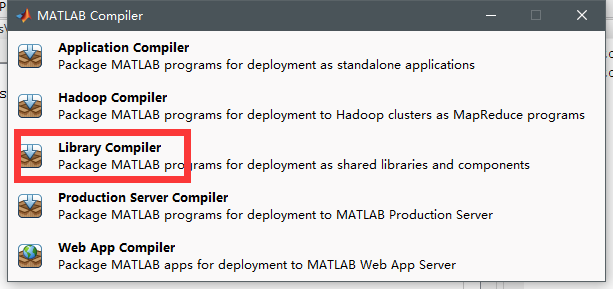3. 第1红框中选择"C++ Shared Library"。第2红框中点击右边的加号，添加刚才编写好的test.m文件。第3红框中输入导出的库的名称。最后点击右上角的"Package"，选择你要输出的路径。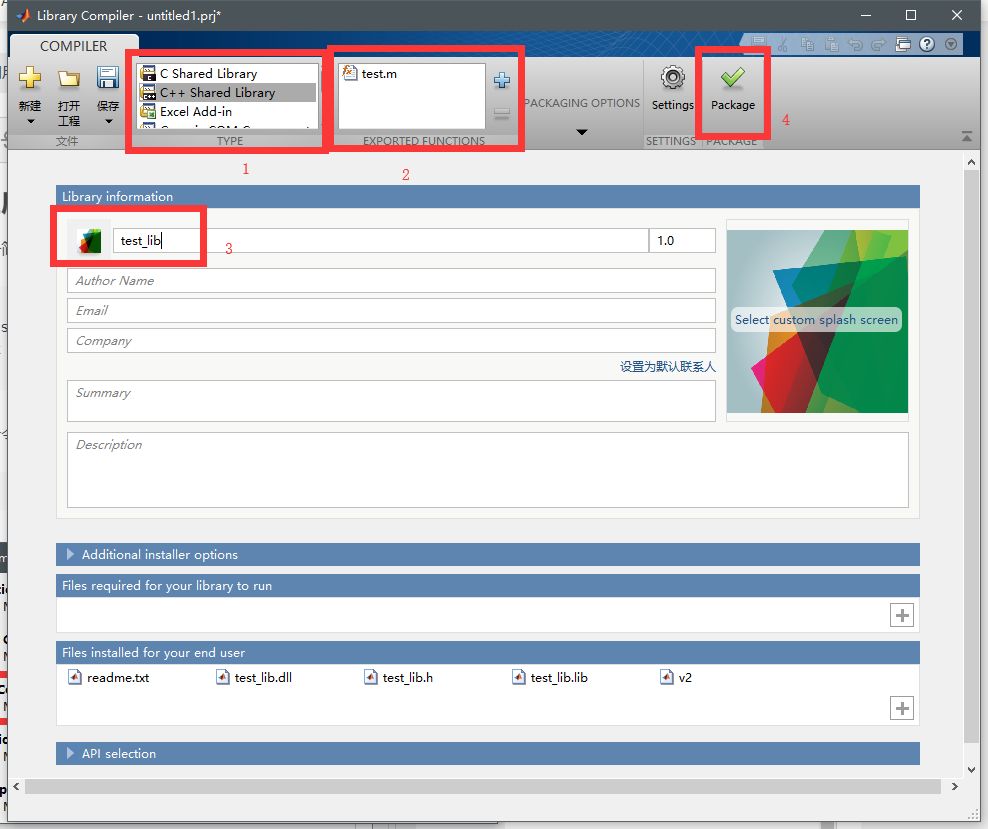4. 最后生成3个文件夹，我们之后要用到“for_redistribution_files_only”里的内容。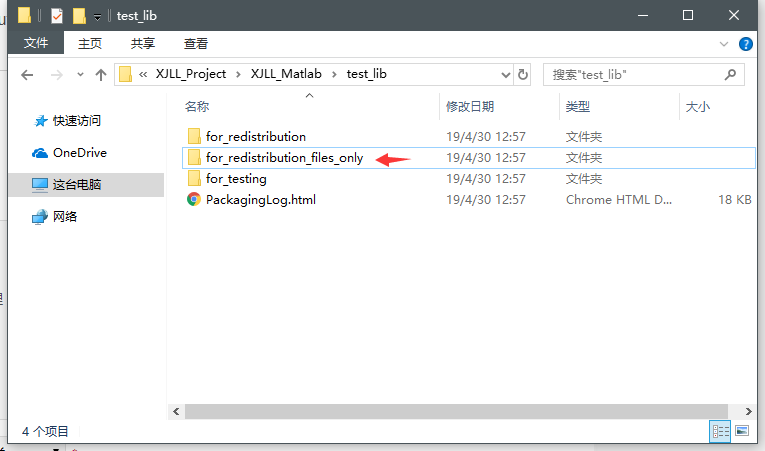在Qt Creator中使用c++库

1. 新建一个Qt项目，项目名称为“XJLL_Qt”，其他保持默认。将“for_redistribution_files_only”文件夹内的“test_lib.dll”，"test_lib.h"，"test_lib.lib"这三个文件拷贝至Qt项目的根目录下：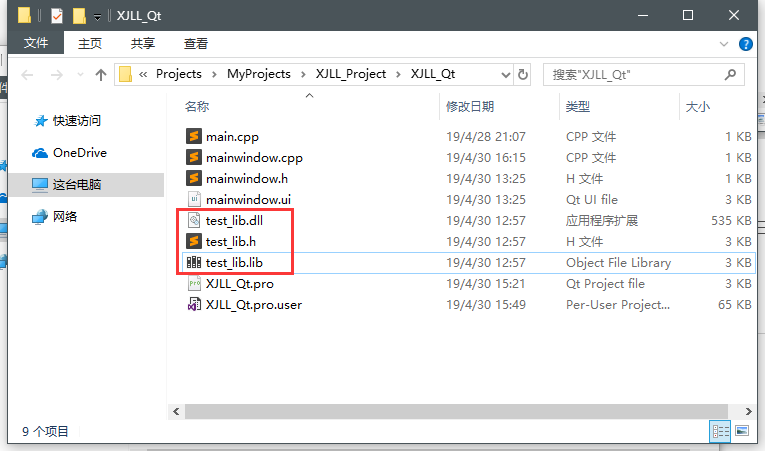2. 在Qt中右键你的项目框中，右键你的项目的根目录，选择“添加现有文件”。选择刚才拷贝过来的"test_lib.h"。

3. 在Qt中右键你的项目框中，右键你的项目的根目录，选择“添加库”，接着选择“外部库”。接着“库文件”选择刚才拷贝过来的“test_lib.lib”，平台只勾选“windows”，下面的都不要勾，然后点击“下一步”并完成添加。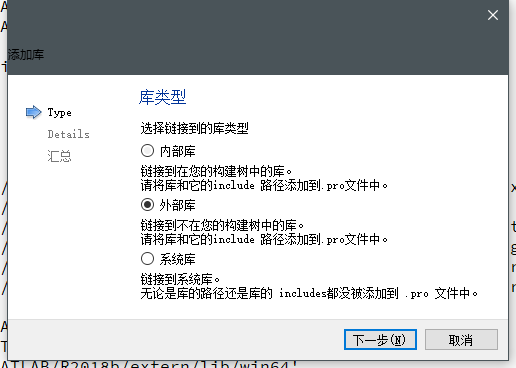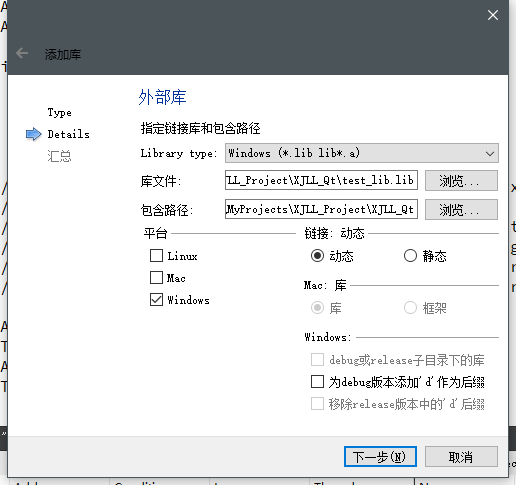4. 打开Qt项目的.pro文件，你会发现多了几行，说明已经成功添加进来了。

win32: LIBS += -L$$PWD/./ -ltest_lib INCLUDEPATH +=$$PWD/.
DEPENDPATH += PWD/.

接着在.pro文件中添加以下几行，其中的matlab路径改成你自己的，当然你也可以像上述操作一样，依次添加这几个库文件，我添加时为了方便，复制粘贴并修改了库名称。

INCLUDEPATH += 'D:/Program Files/MATLAB/R2018b/extern/include'
INCLUDEPATH += 'D:/Program Files/MATLAB/R2018b/extern/include/win64'

win32: LIBS += -L'D:/Program Files/MATLAB/R2018b/extern/lib/win64/microsoft/' -llibmex
win32: LIBS += -L'D:/Program Files/MATLAB/R2018b/extern/lib/win64/microsoft/' -llibmx
win32: LIBS += -L'D:/Program Files/MATLAB/R2018b/extern/lib/win64/microsoft/' -llibmat
win32: LIBS += -L'D:/Program Files/MATLAB/R2018b/extern/lib/win64/microsoft/' -llibeng
win32: LIBS += -L'D:/Program Files/MATLAB/R2018b/extern/lib/win64/microsoft/' -lmclmcr
win32: LIBS += -L'D:/Program Files/MATLAB/R2018b/extern/lib/win64/microsoft/' -lmclmcrrt

INCLUDEPATH += 'D:/Program Files/MATLAB/R2018b/extern/lib/win64/microsoft'
DEPENDPATH += 'D:/Program Files/MATLAB/R2018b/extern/lib/win64/microsoft'
INCLUDEPATH += 'D:/Program Files/MATLAB/R2018b/extern/lib/win64'
DEPENDPATH += 'D:/Program Files/MATLAB/R2018b/extern/lib/win64'


注意每次.pro文件修改后，都要重新执行一遍qmake。（“构建”-->“qmake”）。

5. 简单设置"mainwindow.ui"的界面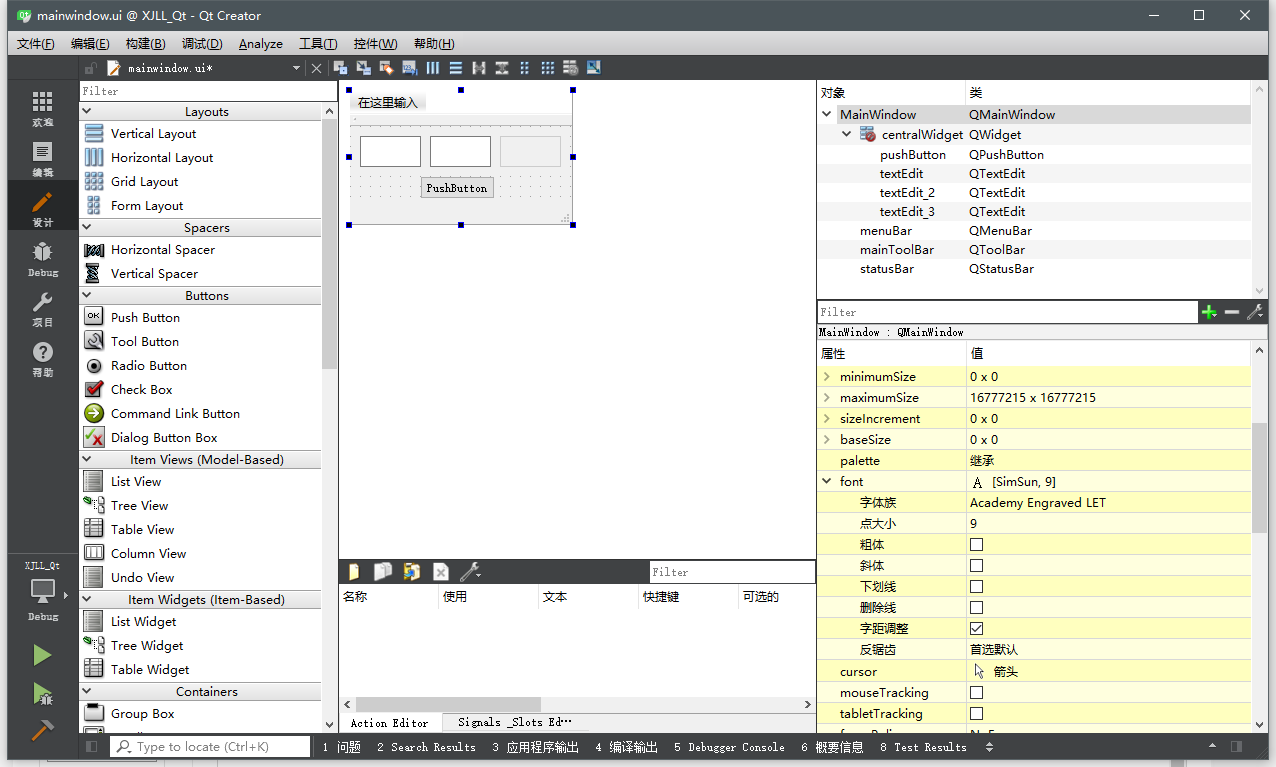6. 在“mainwindow.cpp”引入"test_lib.h"的头文件，“mainwindow.cpp”代码如下：

#include "mainwindow.h"
#include "ui_mainwindow.h"
#include "test_lib.h"  //引入头文件

MainWindow::MainWindow(QWidget *parent) :
QMainWindow(parent),
ui(new Ui::MainWindow)
{
ui->setupUi(this);
}

MainWindow::~MainWindow()
{
delete ui;
}

void MainWindow::on_pushButton_clicked()
{
if(!test_libInitialize()); //不执行test_libInitialize()会报错

mwArray aa(1,1,mxDOUBLE_CLASS,mxREAL);
mwArray bb(1,1,mxDOUBLE_CLASS,mxREAL);
mwArray cc(1,1,mxDOUBLE_CLASS,mxREAL);

double a={ui->textEdit->toPlainText().toDouble()};
double b={ui->textEdit_2->toPlainText().toDouble()};
double c;

aa.SetData(a,1);
bb.SetData(b,1);
test(1,cc,aa,bb);

cc.GetData(c,1);
ui->textEdit_3->setText(QString::number(c));
}


7. 运行你的程序，注意，如果你的matlab是64位的，Qt在构建项目时也要选择64位，图示如下：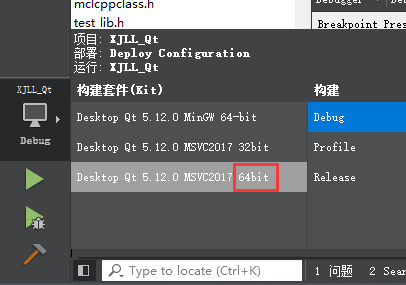8. 最后的运行效果如下：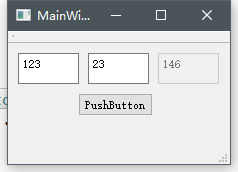疑问

每次运行时，第一次点击按钮会等待较长时间，并且在Qt的“应用程序输出”中有如下输出，不清楚是什么原因。

SetThrowSegvLongjmpSEHFilter(): filter function installed
SetThrowSegvLongjmpSEHFilter(): filter function installed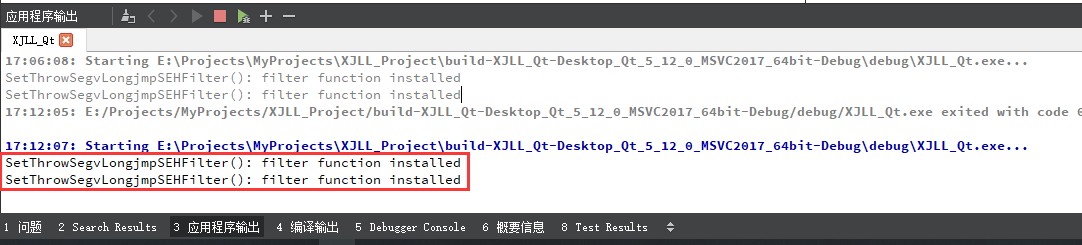展开全文• 完成使用c++调用matlab生成的.dll库函数的小demo 参考了很多人的blog C/C++下调用matlab函数操作说明 C/C++ VS中调用matlab函数的方法 自己动手 按照别人的blog，配置好matlab里边c++编译器配置，我自己用的是qt...
• 首先根据matlab的版本下载对应的matlab运行库matlab 各版本运行库下载地址：...如我下载安装在D...
• c/c++调用matlab生成dll，可以用配置属性的方式。这个需要你的电脑上装matlab，配置路径。参考c调用matlab的dll 加载过程很慢。现在设置项目中需要的库加入。 首先准备需要inlcude的头文件放入一个自己的...
• MATLAB具有很高的计算性能，在该平台上很多算法易于实现，而有时需要使用C++调用Matlab编写的程序，可以将该函数编译成dll库文件，之后在C++中对其进行调用。 本文详细讲解在Matlab中将函数编译成库文件，并在C++中...混合编程
• MATLAB具有很高的计算性能，在该平台上很多算法易于实现，而有时需要使用C++调用Matlab编写的程序，可以将该函数编译成dll库文件，之后在C++中对其进行调用。 本文详细讲解在Matlab中将函数编译成库文件，并在C++中...
• 最近有个任务要对如下图找其中的圆圈，在opencv上面使用cvHoughCircles效果很不好，所以在matlab下试了下imfindcircles函数，发现效果非常...所以问题便转为怎么在c++调用matlab了，我选择的是matlab生成动态链接库d混合编程
• 编译文件： 1、在命令行窗口依次输入以下语句： mex –setup %选择语言 mbuild -setup 选择编译环境，然后进行编译 ...2、使用matlab 工具箱 Library Compiler 点击工具箱，选择c++SharedLibrary，添加待编译的...
• 这里的环境是windows7+vs2010+matlabR2010b上一篇是通过engine来调用matlab中的语句，本篇是通过调用m文件编译成的h/lib/dll文件而实现的。首先写一个函数mysvd.m:1 function [s v d]=mysvd(a)2 [s v d]=svd(a);3 ...
• ## C++调用MatlabDLL应用

千次阅读 2014-12-05 18:29:11
最近在搞组里项目，需要用到C++调用MatlabDLL，在网上查阅了一些资料终于取得一些成果，先总结如下： 首先，对Matlab的m文件进行编译生成C++的DLL与Lib文件。我用到的编译环境为Win7、Matlab2013a、Visual Stdio ...library win7
• 首先强调重要一点，如果你是32位C++程序想要调用matlab生成dll，那么你的matlab需要是32位版本的(网上有说64位matlab也可以转为32位dll，但是本人没有成功，这里就不进行介绍)。同样，如果你是64位C++程序想要调用...
• VS2008 C++ 调用MATLAB 2009b 生成DLL 收藏 刚开始学习用VC++调用matlab生成DLL，找了网上一些资料，难以找到vs2008与MATLAB2009b版本的，按照以往版本做的总是有很多错误。经过两天努力，终于调试成功，这里...
• 在项目中，遇到需要用C++调用Matlab程序来做图像处理，这里记录下整个过程。以供遇到类似问题的读者作参考。此问题棘手的原因主要有在Matlab编写的项目程序中用到了很多图像处理封装库，经过多次尝试，最终在Visual ...qt linux 算法...

# c++调用matlab生成的dllc++ 订阅matlab 订阅